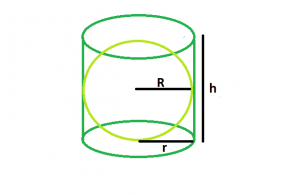# Volume of biggest sphere within a right circular cylinder

Given a right circular cylinder of radiusand height. The task is to find the radius of the biggest sphere that can be inscribed within it.

Examples:

```Input : r = 4, h = 8
Output : 4

Input : r = 5, h= 10
Output :5
```Approach: From the diagram, it is clear that the radius of the sphere will be clearly equal to the base radius of cylinder.

So, R = r

Below is the implementation of the above approach:

## C++

 `// C++ Program to find the biggest sphere ` `// that can be fit within a right circular cylinder ` `#include ` `using` `namespace` `std; ` ` `  `// Function to find the biggest sphere ` `float` `sph(``float` `r, ``float` `h) ` `{ ` ` `  `    ``// radius and height cannot be negative ` `    ``if` `(r < 0 && h < 0) ` `        ``return` `-1; ` ` `  `    ``// radius of sphere ` `    ``float` `R = r; ` `    ``return` `R; ` `} ` ` `  `// Driver code ` `int` `main() ` `{ ` `    ``float` `r = 4, h = 8; ` `    ``cout << sph(r, h) << endl; ` `    ``return` `0; ` `} `

## Java

 `// Java Program to find the biggest ` `// sphere that can be fit within a  ` `// right circular cylinder ` `import` `java.io.*; ` ` `  `class` `GFG  ` `{ ` ` `  `// Function to find the biggest sphere ` `static` `float` `sph(``float` `r, ``float` `h) ` `{ ` ` `  `    ``// radius and height cannot  ` `    ``// be negative ` `    ``if` `(r < ``0` `&& h < ``0``) ` `        ``return` `-``1``; ` ` `  `    ``// radius of sphere ` `    ``float` `R = r; ` `    ``return` `R; ` `} ` ` `  `// Driver code ` `public` `static` `void` `main (String[] args)  ` `{ ` `    ``float` `r = ``4``, h = ``8``; ` `    ``System.out.println(sph(r, h)); ` `} ` `} ` ` `  `// This code is contributed  ` `// by inder_verma `

## Python3

 `# Python 3 Program to find the biggest  ` `# sphere that can be fit within a right ` `# circular cylinder ` ` `  `# Function to find the biggest sphere ` `def` `sph(r, h): ` `     `  `    ``# radius and height cannot  ` `    ``# be negative ` `    ``if` `(r < ``0` `and` `h < ``0``): ` `        ``return` `-``1` ` `  `    ``# radius of sphere ` `    ``R ``=` `r ` `    ``return` `float``(R) ` ` `  `# Driver code ` `r, h ``=` `4``, ``8` `print``(sph(r, h)) ` ` `  `# This code is contributed  ` `# by PrinciRaj1992 `

## C#

 `// C# Program to find the biggest ` `// sphere that can be fit within a  ` `// right circular cylinder ` `using` `System; ` ` `  `class` `GFG  ` `{ ` ` `  `// Function to find the biggest sphere ` `static` `float` `sph(``float` `r, ``float` `h) ` `{ ` ` `  `    ``// radius and height cannot  ` `    ``// be negative ` `    ``if` `(r < 0 && h < 0) ` `        ``return` `-1; ` ` `  `    ``// radius of sphere ` `    ``float` `R = r; ` `    ``return` `R; ` `} ` ` `  `// Driver code ` `public` `static` `void` `Main ()  ` `{ ` `    ``float` `r = 4, h = 8; ` `    ``Console.WriteLine(sph(r, h)); ` `} ` `} ` ` `  `// This code is contributed  ` `// by shs.. `

## PHP

 ` `

Output:

```4
```

Attention reader! Don’t stop learning now. Get hold of all the important DSA concepts with the DSA Self Paced Course at a student-friendly price and become industry ready.

My Personal Notes arrow_drop_upBudding Web DeveloperKeen learnerAverage CoderDancer&Social Activist

If you like GeeksforGeeks and would like to contribute, you can also write an article using contribute.geeksforgeeks.org or mail your article to contribute@geeksforgeeks.org. See your article appearing on the GeeksforGeeks main page and help other Geeks.

Please Improve this article if you find anything incorrect by clicking on the "Improve Article" button below.